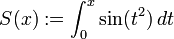# Fresnel sine integral

(diff) ← Older revision | Latest revision (diff) | Newer revision → (diff)
For functions involving angles (trigonometric functions, inverse trigonometric functions, etc.) we follow the convention that all angles are measured in radians. Thus, for instance, the angle of$90\,^\circ$ is measured as$\pi/2$.
The Fresnel sine integral, which we here denote by$S$, is defined as:$S(x) := \int_0^x \sin(t^2) \, dt$